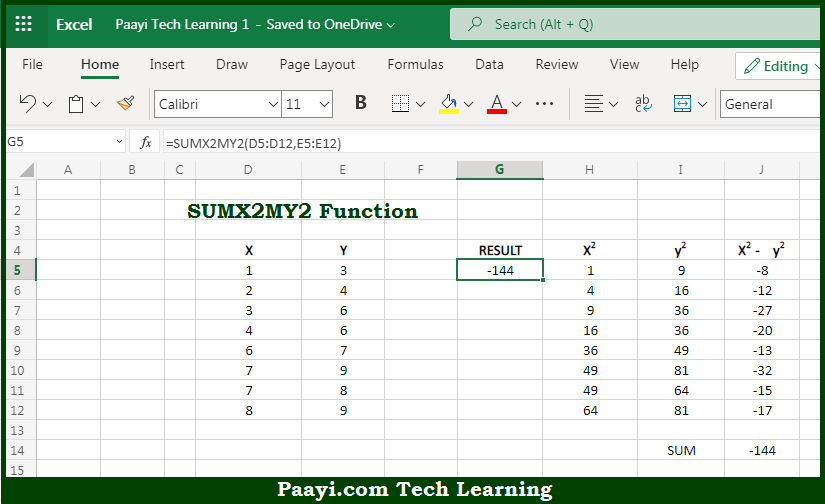# Learn How to Use Microsoft Excel SUMX2MY2 Function

Written by | 0 Comments | 431 Views

In this article, you will learn how to use the Microsoft Excel SUMX2MY2 function and its prime function in Microsoft Excel. You will also get to know the Microsoft Excel SUMX2MY2 function return value and syntax with the help of some examples.

Microsoft Excel SUMX2MY2 Function

The main function of the Microsoft Excel SUMX2MY2 function is to get the sum of the difference of squares in two arrays. That implies, with the help of the SUMX2MY2 function you can able to get a sum of the difference of squares corresponding to the values n two arrays. It should be noted that the values that you provided can be in the form of constants, cell references, or ranges. So, with the help of the SUMX2MY2 function, you can able to get the sum of the difference of squares in two arrays.

Return Value of SUMX2MY2 Function

The return value will be the calculated sum of the difference of squares.

Syntax of SUMX2MY2 Function

=SUMX2MY2(array-x, array-y)

Where the arguments:

• array-x: This is the first range or array containing numeric values.
• array-y: This is the second range or array containing numeric values (optional).

## How to Use Microsoft Excel SUMX2MY2 Function?So we know that Microsoft Excel SUMX2MY2 function you can able to get the sum of the difference of squares in two arrays. That implies, with the help of the SUMX2MY2 function you can able to get the sum of the difference of squares corresponding to the values n two arrays. It should be noted that the values that you provided can be in the form of constants, cell references, or ranges. So, with the help of the SUMX2MY2 function, you can able to get the sum of the difference of squares in two arrays.